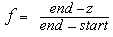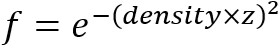# glFogiv function

The glFogfv function specifies fog parameters.

## Syntax

``````void WINAPI glFogiv(
GLenum pname,
const GLint  *params
);
``````

## Parameters

pname

Specifies a fog parameter.

Accepts one of the following values.

Value Meaning
GL_FOG_MODE
The params parameter is a single integer value that specifies the equation to be used to compute the fog blend factor, f. Three symbolic constants are accepted: GL_LINEAR, GL_EXP, and GL_EXP2. The equations corresponding to these symbolic constants are defined in the following Remarks section. The default fog mode is GL_EXP.
GL_FOG_DENSITY
The params parameter is a single integer value that specifies density, the fog density used in both exponential fog equations. Only nonnegative densities are accepted. The default fog density is 1.0.
GL_FOG_START
The params parameter is a single integer value that specifies start, the near distance used in the linear fog equation. The default near distance is 0.0.
GL_FOG_END
The params parameter is a single integer value that specifies end, the far distance used in the linear fog equation. The default far distance is 1.0.
GL_FOG_INDEX
The params parameter is a single integer value that specifies if , the fog color index. The default fog index is 0.0.
GL_FOG_COLOR
The params parameter contains four integer or floating-point values that specify Cf , the fog color. Integer values are mapped linearly such that the most positive representable value maps to 1.0, and the most negative representable value maps to -1.0. Floating-point values are mapped directly. After conversion, all color components are clamped to the range [0,1]. The default fog color is (0,0,0,0).

params

Specifies the value or values to be assigned to pname. GL_FOG_COLOR requires an array of four values. All other parameters accept an array containing only a single value.

## Return value

This function does not return a value.

## Error codes

The following error codes can be retrieved by the glGetError function.

Name Meaning
GL_INVALID_ENUM
pname was not an accepted value.
GL_INVALID_OPERATION
The function was called between a call to glBegin and the corresponding call to glEnd.

## Remarks

You enable and disable fog with glEnable and glDisable, using the argument GL_FOG. While enabled, fog affects rasterized geometry, bitmaps, and pixel blocks, but not buffer-clear operations.

The glFogiv function assigns the value or values in params to the fog parameter specified by pname.

Fog blends a fog color with each rasterized pixel fragment's posttexturing color using a blending factor f. Factor f is computed in one of three ways, depending on the fog mode. Let z be the distance in eye coordinates from the origin to the fragment being fogged. The equation for GL_LINEAR fog is:The equation for GL_EXP fog is:The equation for GL_EXP2 fog is:Regardless of the fog mode, f is clamped to the range [0,1] after it is computed. Then, if OpenGL is in RGBA color mode, the fragment's color Cr is replaced byIn color-index mode, the fragment's color index ir is replaced byThe following functions retrieve information related to the glFog functions:

glGet with argument GL_FOG_COLOR

glGet with argument GL_FOG_INDEX

glGet with argument GL_FOG_DENSITY

glGet with argument GL_FOG_START

glGet with argument GL_FOG_END

glGet with argument GL_FOG_MODE

glIsEnabled with argument GL_FOG

## Requirements

Requirement Value
Minimum supported client
Windows 2000 Professional [desktop apps only]
Minimum supported server
Windows 2000 Server [desktop apps only]
Gl.h
Library
Opengl32.lib
DLL
Opengl32.dll